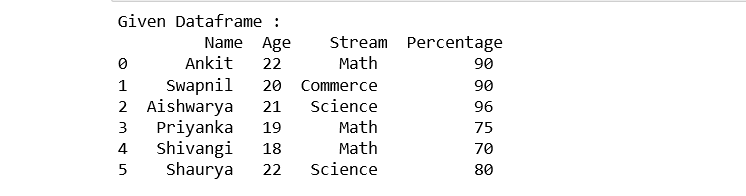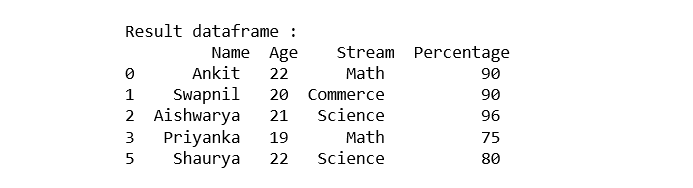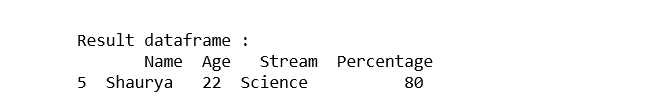# Ways to filter Pandas DataFrame by column values

Filtering a Pandas DataFrame by way of column values is a commonplace operation while running with information in Python. You can use various methods and techniques to achieve this. Here are numerous ways to filter out a Pandas DataFrame through column values.

In this post, we will see different ways to filter Pandas Dataframe by column values. First, Let’s create a Dataframe:

## Python3

 `# importing pandas ` `import` `pandas as pd ` `  `  `# declare a dictionary` `record ``=` `{ `   ` ``'Name'` `: [``'Ankit'``, ``'Swapnil'``, ``'Aishwarya'``, ` `          ``'Priyanka'``, ``'Shivangi'``, ``'Shaurya'` `],` `  `  ` ``'Age'` `: [``22``, ``20``, ``21``, ``19``, ``18``, ``22``], ` `  `  ` ``'Stream'` `: [``'Math'``, ``'Commerce'``, ``'Science'``, ` `            ``'Math'``, ``'Math'``, ``'Science'``], ` `  `  ` ``'Percentage'` `: [``90``, ``90``, ``96``, ``75``, ``70``, ``80``] } ` `  `  `# create a dataframe ` `dataframe ``=` `pd.DataFrame(record,` `                         ``columns ``=` `[``'Name'``, ``'Age'``, ` `                                    ``'Stream'``, ``'Percentage'``]) ` `# show the Dataframe` `print``(``"Given Dataframe :\n"``, dataframe)`

Output:### Selecting rows of Pandas Dataframe based on particular column value using ‘>’, ‘=’, ‘=’, ‘<=’, ‘!=’ operator.

Example 1: Selecting all the rows from the given Dataframe in which ‘Percentage’ is greater than 75 using [ ].

## Python3

 `# selecting rows based on condition ` `rslt_df ``=` `dataframe[dataframe[``'Percentage'``] > ``70``] ` `  `  `print``(``'\nResult dataframe :\n'``, rslt_df)`

Output:Example 2: Selecting all the rows from the given Dataframe in which ‘Percentage’ is greater than 70 using loc[ ]

## Python3

 `# selecting rows based on condition ` `rslt_df ``=` `dataframe.loc[dataframe[``'Percentage'``] > ``70``] ` `  `  `print``(``'\nResult dataframe :\n'``, ` `      ``rslt_df)`

Output:### Selecting those rows of Pandas Dataframe whose column value is present in the list using isin() method of the dataframe.

Example 1: Selecting all the rows from the given dataframe in which ‘Stream’ is present in the options list using [ ].

## Python3

 `options ``=` `[``'Science'``, ``'Commerce'``] ` `  `  `# selecting rows based on condition ` `rslt_df ``=` `dataframe[dataframe[``'Stream'``].isin(options)] ` `  `  `print``(``'\nResult dataframe :\n'``,` `      ``rslt_df)`

Output:Example 2: Selecting all the rows from the given dataframe in which ‘Stream’ is present in the options list using loc[ ].

## Python

 `options ``=` `[``'Science'``, ``'Commerce'``] ` `  `  `# selecting rows based on condition ` `rslt_df ``=` `dataframe.loc[dataframe[``'Stream'``].isin(options)] ` `  `  `print``(``'\nResult dataframe :\n'``, ` `      ``rslt_df)`

Output:### Selecting rows of  Pandas Dataframe based on multiple column conditions using ‘&’ operator.

Example1: Selecting all the rows from the given Dataframe in which ‘Age’ is equal to 22 and ‘Stream’ is present in the options list using [ ].

## Python3

 `options ``=` `[``'Commerce'` `,``'Science'``] ` `  `  `# selecting rows based on condition ` `rslt_df ``=` `dataframe[(dataframe[``'Age'``] ``=``=` `22``) & ` `          ``dataframe[``'Stream'``].isin(options)] ` `  `  `print``(``'\nResult dataframe :\n'``,` `      ``rslt_df)`

Output:Example 2: Selecting all the rows from the given Dataframe in which ‘Age’ is equal to 22 and ‘Stream’ is present in the options list using loc[ ].

## Python3

 `options ``=` `[``'Commerce'``, ``'Science'``] ` ` `  `# selecting rows based on condition ` `rslt_df ``=` `dataframe.loc[(dataframe[``'Age'``] ``=``=` `22``) & ` `              ``dataframe[``'Stream'``].isin(options)] ` `  `  `print``(``'\nResult dataframe :\n'``,` `      ``rslt_df)`

Output:Whether you're preparing for your first job interview or aiming to upskill in this ever-evolving tech landscape, GeeksforGeeks Courses are your key to success. We provide top-quality content at affordable prices, all geared towards accelerating your growth in a time-bound manner. Join the millions we've already empowered, and we're here to do the same for you. Don't miss out - check it out now!

Previous
Next